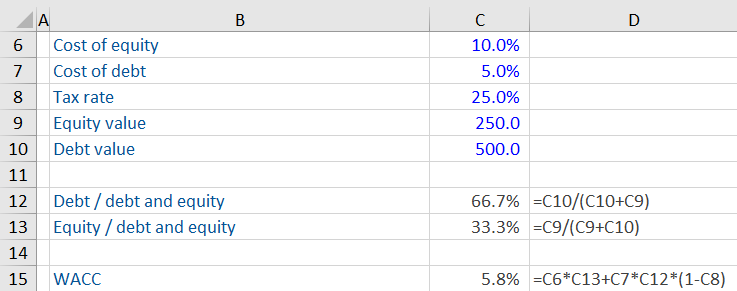## What is the Weighted Average Cost of Capital?

The weighted average cost of capital or WACC is the return required by capital providers to compensate for the risk profile of investing in a company.

The forecast cash flows and the terminal value in a DCF valuation model are discounted to present value using a discount rate that is commensurate with the risk associated with their generation. Since the financing of the operational business is provided by both debt and equity then the discount rate used is the weighted average cost of capital or WACC.

## Key Learning Points

• The weighted average cost of capital (WACC) is the required rate of return by capital providers to compensate for the risk profile of the underlying asset
• The return is dependent on two key factors: the cost of financing (debt and equity) and the proportion of each used by the business to fund its operations and growth
• The capital asset pricing model (CAPM) is a common method used to calculate the cost of equity (required rate of return to equity holders)
• Cost of debt is calculated using publicly traded debt as we want to use the most recent and up to date information
• WACC is used in a discounted cash flow analysis and the valuation is very sensitive to WACC

## Formula

The weighted average cost of capital can be summarized as follows:

WACC = ((Cost of debt * Proportion of debt) + (Cost of equity * Proportion of equity))

Cost of net debt and especially the cost of equity are difficult to accurately calculate.## Free Cash Flows

The weighted average cost of capital reflects the return required by the providers of capital to compensate for the risk profile of the underlying asset. Therefore, it is a market-based measure. The components of the capital structure included in WACC should also be consistent with items considered to be financing when calculating free cash flow.

• Calculate consistently with FCF construction
• Use market values
• Include all capital providers